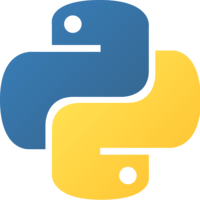This lesson is for members. Join us? Get access to all 3,000+ tutorials + a community with expert developers around the world.

Unlock This Lesson
1×
Become a member
to unlock all featuresLevel Up!

Access all courses & lessons on egghead today and lock-in your price for life.

Autoplay

Make Predictions on New Data with a Multi Category Classification Networkpython
^3.0.0

Once we have built a multi-class classification network, we'll use it to make predictions on new data that wasn't used during training. We'll start by calling the predict method, which returns the probability that each input data row belongs to each one of the possible classes. Then, we'll use the predict_classes method to only output the class prediction as an integer, which might be easier to use in a production system.CodeYou must be a Member to view code

Access all courses and lessons, track your progress, gain confidence and expertise.

Become a Member
and unlock code for this lessonDiscussTranscript

Instructor: We've defined our model for multiclass classification, and then fit it on data that we've pulled in from a CSV. After we fit our network, we would like to use that model to make predictions on new data.

Let's make some new data by making a Numpy array with three new data rows. This is new data that the model hasn't seen yet. I've picked one new data row for each class of data that we have. The first row is class zero, which is Iris setosa.

The second row should be class one, which is Iris versicolor. The third row is an example of class two, which is Iris virginica. Now, we can make a class prediction for each row by calling the predict method on the model, and pass in the data that we want to make predictions on.

Then we can print the output with a blank line, just for formatting. When we rerun that, the model trains like normal. Then we see predictions each for the three rows that we just created. You might notice that the values are all scientific notation, and that can be difficult to read.

Let's clean that up by telling Numpy to suppress scientific notation, by calling set_printoptions on Numpy, and set suppress equal to true. Then we can run that again, and now, the output is easier to read.

To interpret this output, remember that the input was made into categorical data by calling to_categorical with the data, which turns it from an integer like zero, one, or two into a one hot encoded value.

What we're seeing on the output is the probability that each of the inputs belongs to each one of the classes. It's also one hot encoded. Each row is the row of our input data, and each column represents one of the possible classes.

If you were to round these values, you can see that the first value would have a one in the index zero spot, and a zero in the two other spots, which means the prediction for this row is class zero, or Iris setosa.

For the second row, the highest value is in the index one spot, and for the last row, the highest value is in the index two spot, which means our network is correctly predicting those as well. This can be confusing to read and deal with, however.

If you don't care about the probability for each of the output classes, you can just see the class predictions by calling the predict_classes method, instead of just the predict method.

If we add a line to call predict_classes, and rerun that, we can see that the output is just the class numbers that are being predicted, which match up with the one hot encoded output that we saw before, but it may be easier to interpret and use.